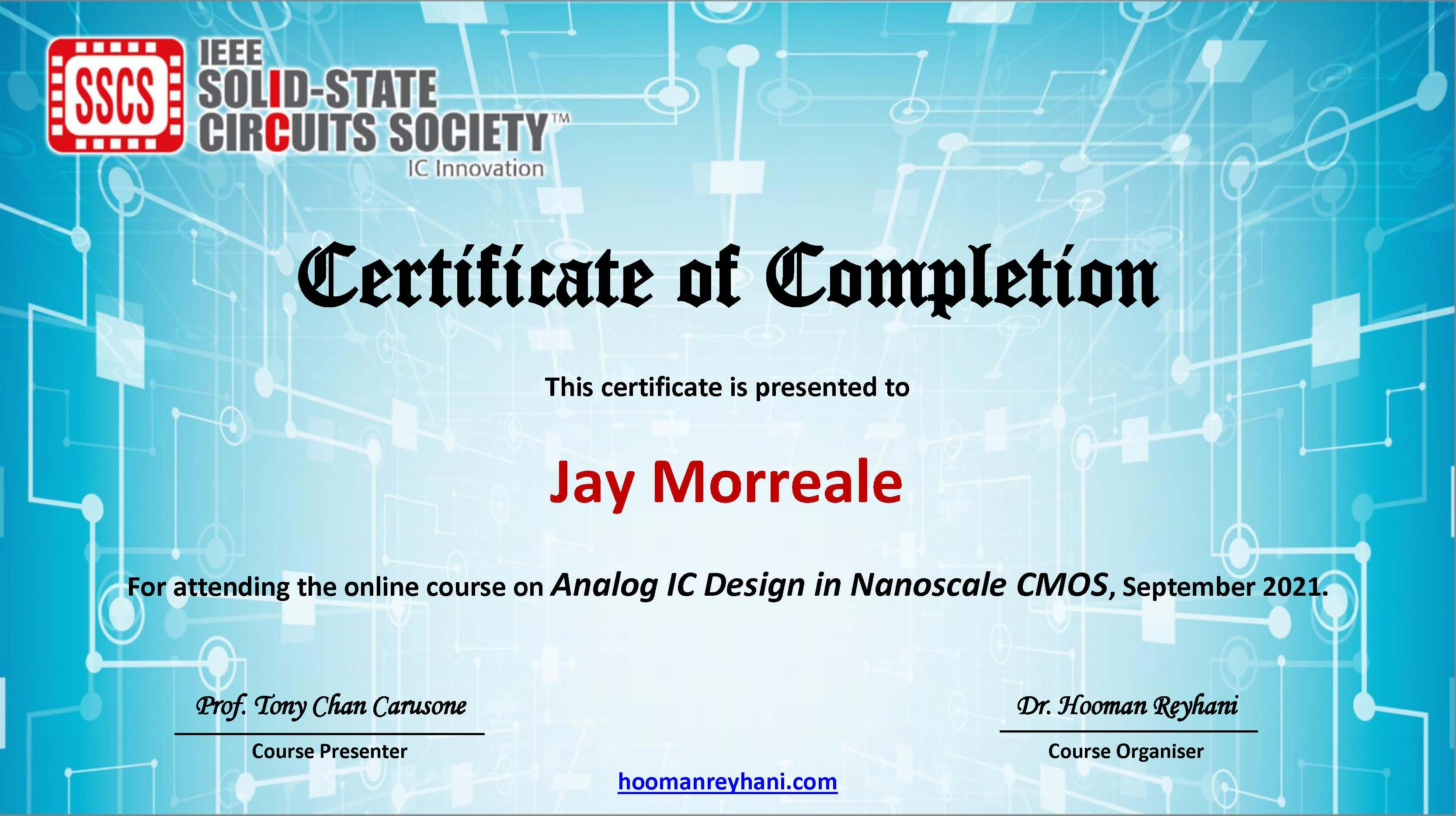## Analog IC Design Course Homework 1 SIMetrix Edition

morreale Monday 11 of October, 2021
The Analog IC Design in Nanoscale CMOS short course was held September 7 to October 1, 2021 virtually from the University of Limerick and taught by Professor Tony Chan Carusone. As part of the course, an eight week license to the SIMetrix Technologies SPICE simulator was provided and was used to find solutions to the optional homework problems provided in the class. Here, I show how I used the simulator and the methods presented in the course to find solutions to the problems for homework assignment 1. Homework 1 contains problems 1, 2 and a bonus problem. For more information about the course see Analog IC Design in Nanoscale CMOS Short Course.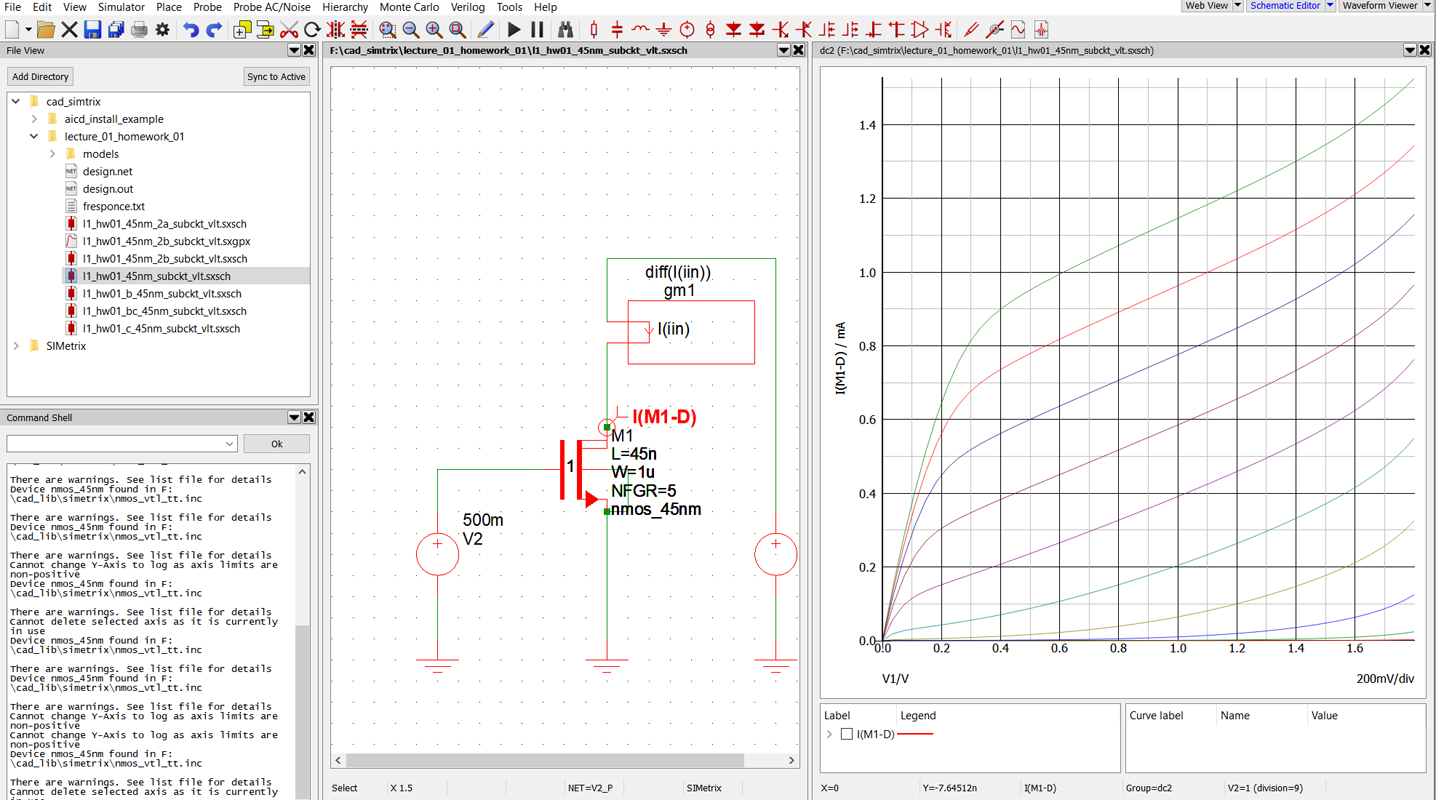For homework 1, we were asked to generate the IV curves for Id vs VDS, Id vs VGS, and gm vs VGS, find the subthreshold slope using Id and gm, and find the unity gain bandwidth for nanoscale transistors using the 45 nm device models with channel lengths of 45 and 90 nm.

Models for 45 nm and 7 nm process transistors were supplied on the course website. The 45 nm models included the low threshold High-speed (vtl) NMOS and PMOS for the typical NMOS and typical PMOS (TT) transistor process variations. The 7 nm models are used in future homework assignments.

## Problem 1: Using 45nm device models

### 1a. plot I‐V characteristics

A schematic was created to plot the IV curves of the transistor using the NMOS_45nm model with length L=45nm, width W=1u, and Number of Fingers NFGR=5. Voltage sources VGS and VDS were added to provide the gate voltage VGS and the drain-source voltage VDS, respectively, to generate the IV-curves.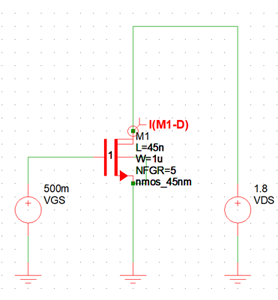The model files were added to the SIMetrix model library by dropping them on to the command shell window. The NMOS_45nm model symbol was placed on the on the schematic from the Select Device panel using Place -> From Model Library -> Recently added models.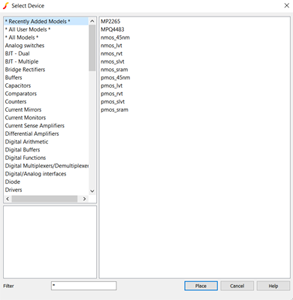A current probe was added to measure Id. The probe was placed using Place -> Probe -> Current Probe and then left clicking on the drain pin of the M1. The Simulate -> Choose Analysis set up was configured for a multistep DC sweep of VDS and VGS as shown below.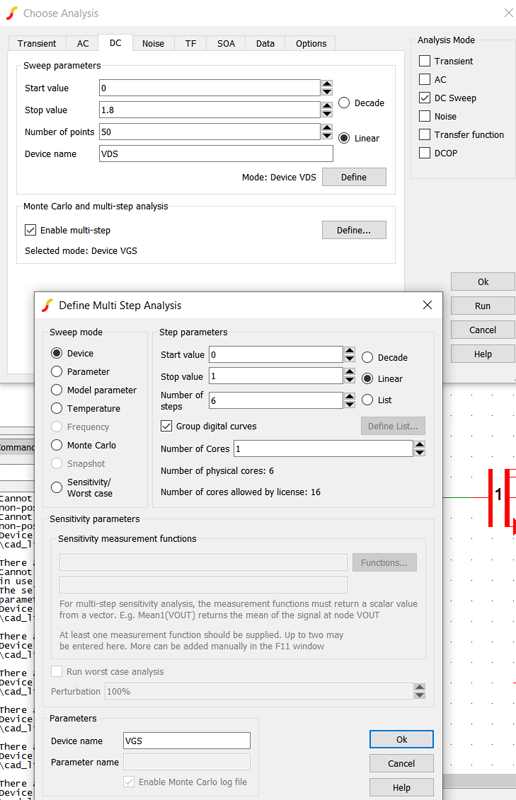The IV curve of the NMOS_45nm device is shown for VDS from 0 to 1.8 V, and VGS from 0 to 1 V in steps of 200 mV.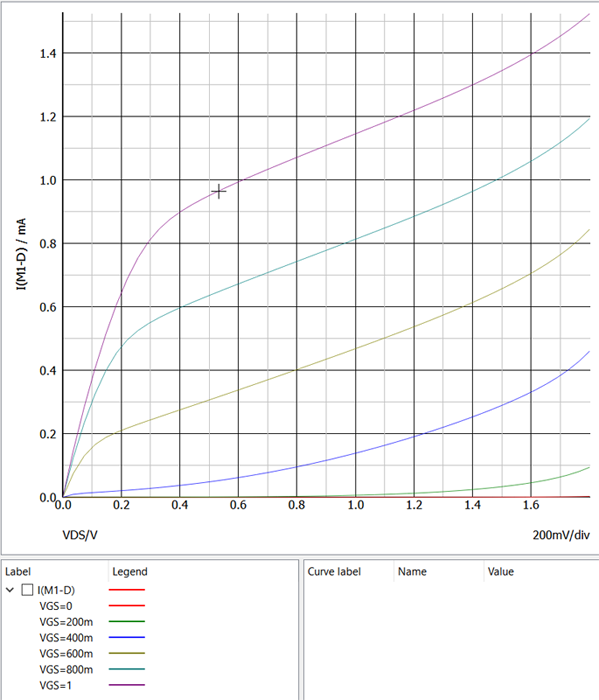### 1b. Extract Vt with VDS = 10mV (extrapolating linear part of the Id vs VGS plot)

With VDS = 10 mV, the transistor operates in the triode region. The circuit from above was modified by replacing VDS with a battery set to 10 mV and the DC Sweep was set to a single sweep VGS (VGS) from 0 to 800 mV.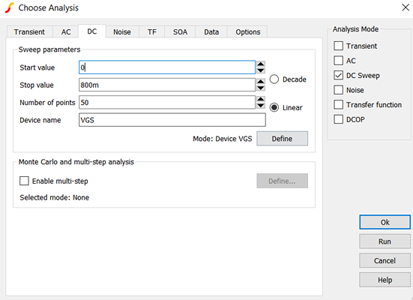The revised schematic is shown below.An arbitrary probe was added and connected in series with the drain of M1 to measure Id and compute gm using the diff() function. The diff() function returns the derivative of the current I(iin) with respect to the main sweep variable VGS yielding
$$\frac{\partial I_d}{\partial V_{GS}}$$
which is gm. This probe was added using Probe -> Create and Place Arbitrary Probe and it was set up as shown in the figure below. The Axis Type -> Use dedicated plot was selected so that curve appears on its own grid (plot).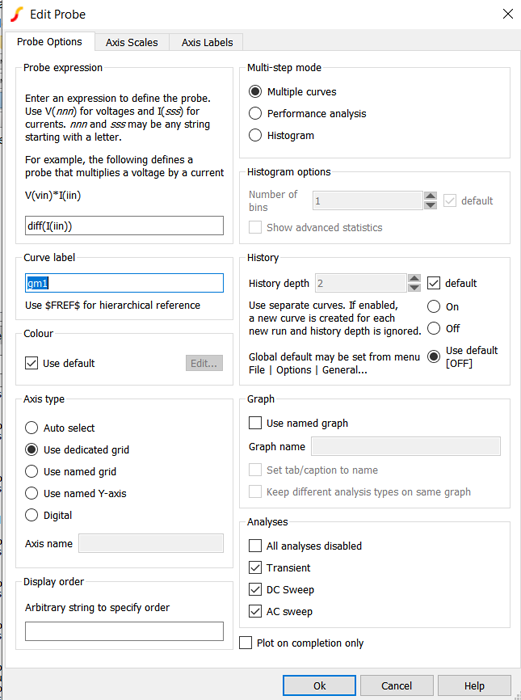gm (top plot) and Id (bottom plot) vs VGS are shown in the plot below.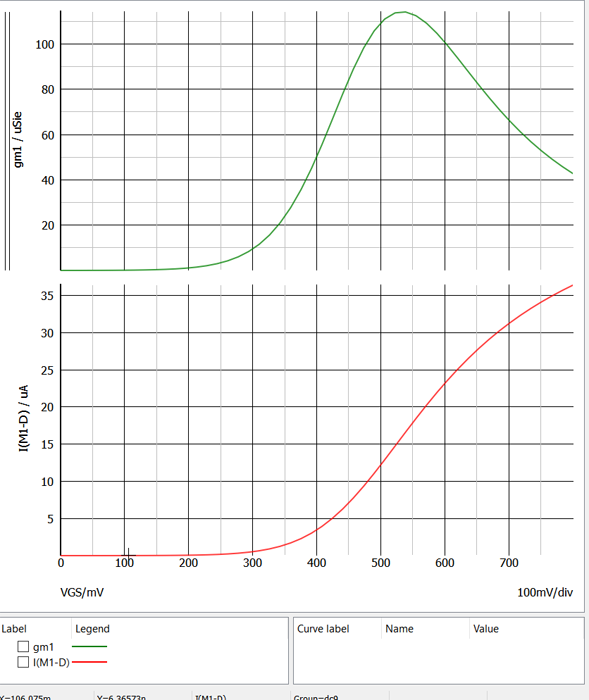Using Id vs VGS, Vt was found by projecting the linear region to the VGS axis starting from the peak in the gm vs VGS curve. Vt is estimated to be around 390 mV.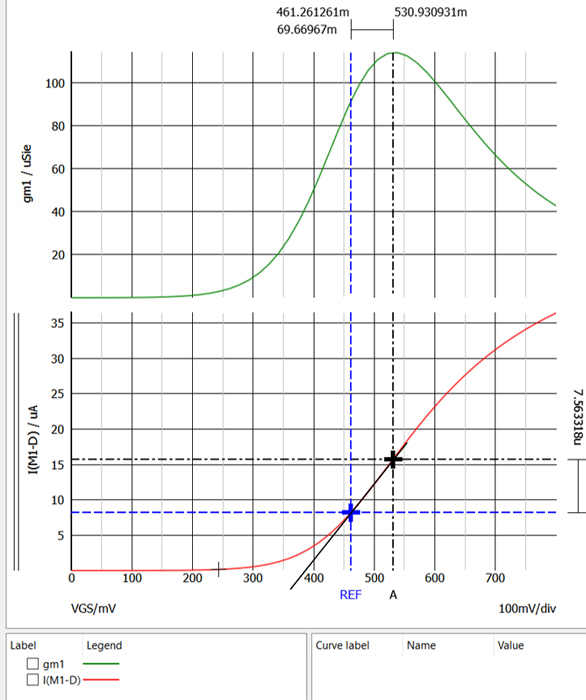### 1c. Extract Vt with VDS = 0.8V (extrapolating the linear part of the gm vs. VGS plot).

The schematic was modified by setting the battery (VDS) to 800 mV and is shown below.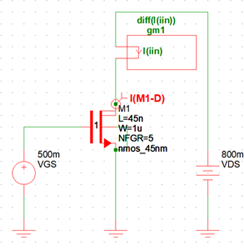In the bottom IV curve shown below, Id is linear for VGS > 450 mV indicating that the transistor is operating in the active region. Using gm vs VGS (top plot), Vt was found by projecting the linear region starting from the linear part of the curve to the VGS axis. Vt is estimated to be about 270 mV.### 1d. Compare results from parts (b) and (c ). What's the difference? What might explain this?

The threshold voltage Vt=390 mV at VDS=10 mV lowers to Vt=270 mV at VDS=800 mV due to the higher drain-source voltage (VDS) and is a result of Drain Induced Barrier Lowering (DIBL).

At VDS=10 mV, the transistor operates in triode mode where
$$I_d=\mu_n C_{ox} (\frac{W}{L})V_{eff}V_{DS}$$
At VDS=800 mV, the transistor operates in the active/saturation region/mode where
$$I_d=\frac{1}{2}\mu_n C_{ox} (\frac{W}{L})V_{eff}^2$$
~181~n is the nmos carrier mobility, COX is the gate capacitance, W is the channel width, L is the channel Length, Veff is the effective gate voltage (VGS-Vt), and VDS is the drain-source voltages.

### 1e. Plot I‐V characteristics on a semilog scale. What is the subthreshold slope? Is GIDL evident?

The simulation was modified to show gm and Id vs VGS for both VDS voltages of 10 mV and 800 mV. The Choose Analysis panels were configure as shown below.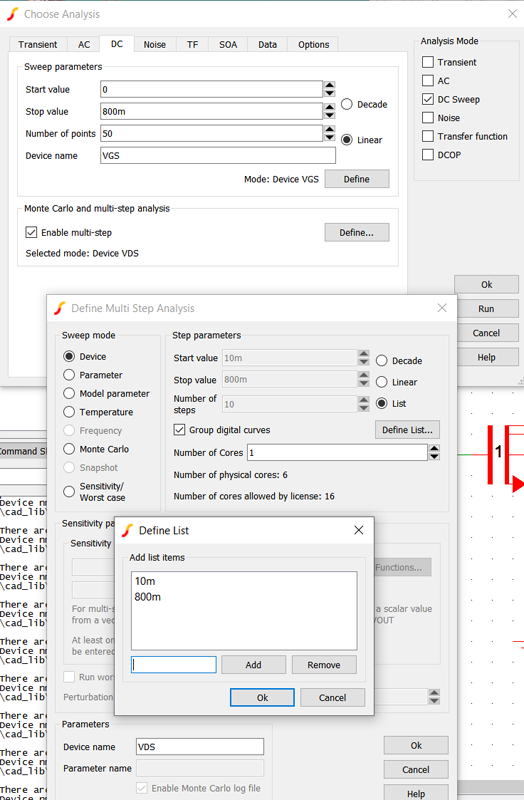gm vs VGS is shown in the top plot and Id vs VGS is shown in the bottom plot. For both plots, VDS = 800 mV is the green trace and VDS = 10 mV is the red trace.Using the Id vs VGS at VDS=800 mV, the subthreshold slope is found to be 100.5 mV/decade. The cursors were dragged to place them a decade a part in the linear part of the current curve to easily determine the subthreshold slope in mV/decade from the voltage difference between the cursors at the top of the plot.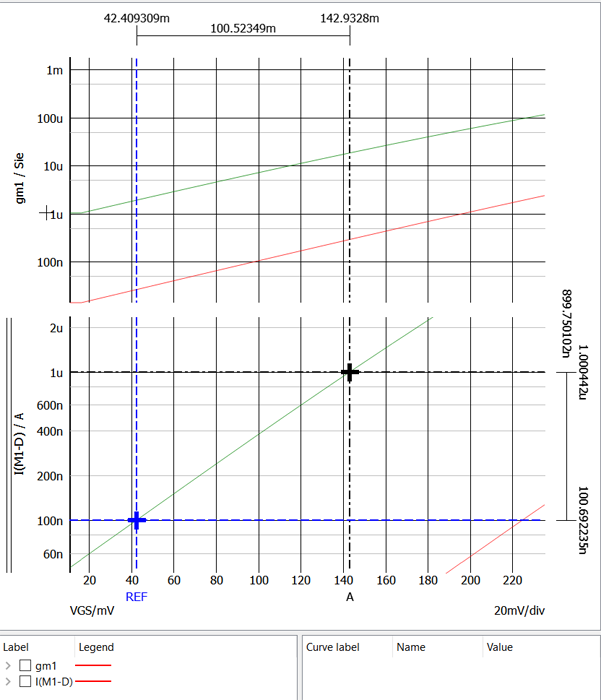Gate Induced Drain Leakage (GIDL) isn't present in this transistor because Id doesn't increase at low VGS voltages.

### 1f. Plot gm/ID vs. VGS (with VDS = 0.8V). How does the value of obtained from plot (i.e. in subthreshold) compare with the subthreshold slope in part (e)?

gm/ID vs VGS was added to the plot using the Add Curve button and the curve configuration is shown below. m1#d is the Define Curve notation for the drain current Id of transistor M1, and diff() is the derivative function.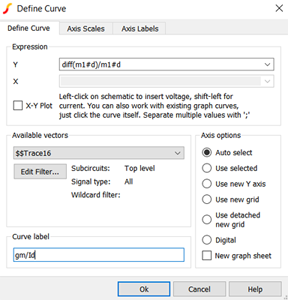Then 1/diff(log10(m1#d)) was added to a grid to plot gm/ID vs. VGS so that the subthreshold slope could be determined using the cursor measurements. The curve is plotted on a new grid by selecting Use new grid.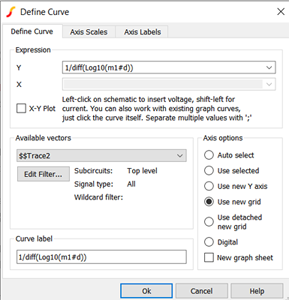The new curve is shown below and can be used to determine n using
$$\frac{g_m}{I_d} = \frac{q}{n k T}$$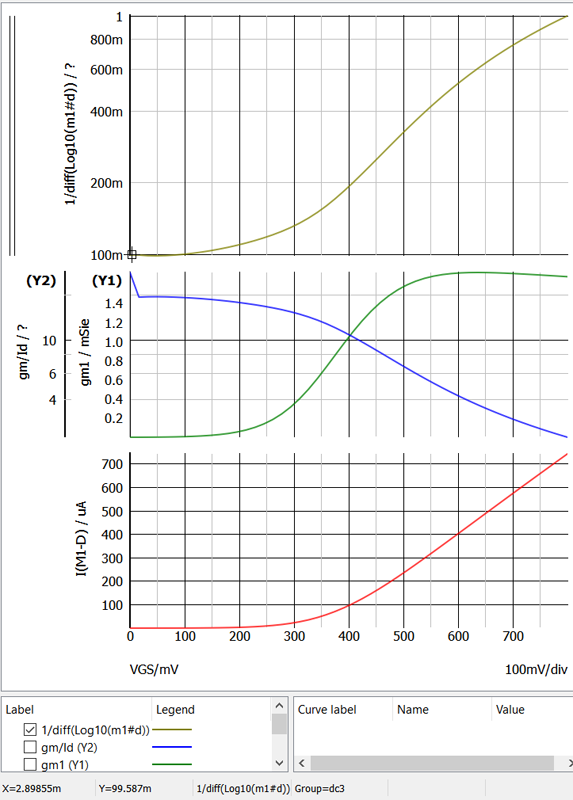The subthreshold slope is 99.587 mV/decade using this method (see y=99.587m at the bottom of the figure). n=1.67 was found using
$$Ln(10)\frac{nKT}{q}=subthreshold slope$$
That is
$$n=\frac{99.587 mV}{(2.3kT/q)}$$

## Problems 2: Also using 45nm device models

### 2a. Find intrinsic gain of a transistor with L=45nm. What operating point maximizes the gain? How does this change with L=90nm?

The calculations for the intrinsic gain |Ai| and rds are shown below.
$$\frac {1}{r_{ds}}=g_{ds}=\frac{\partial I_d}{\partial V_{DS}}=\lambda I_d$$
$$|A_i|=g_m r_{ds}$$
The intrinsic gain was extracted from the AC analysis plot and was found to be 5.03 for the L=45 nm NMOS transistors and the 27.38 for the L=90 nm NMOS transistor at 10 MHz. Notice that the transistor with the shorter length (L=45 nm) has lower gain and more bandwidth, and the transistor with the longer channel length (L=90 nm) has more gain and lower bandwidth (see Carusone et. al. pg. 38).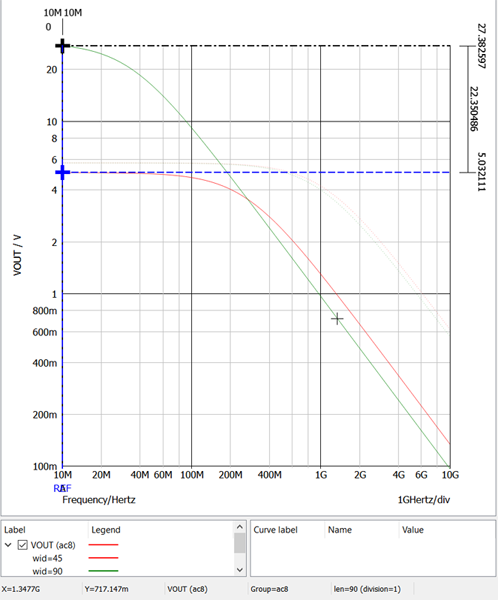### 2b. What is the maximum unity‐gain bandwidth achievable when driving a load capacitance of 200 fF with a common‐source transistor while consuming 0.5mW from a 1‐V supply?

The schematic shows the nmos_45nm transistor with a bias circuit of L1 and C2 driven by a 1 V AC source. C1 is the 200 fF load and I1 is the 500 ~181~A supply current.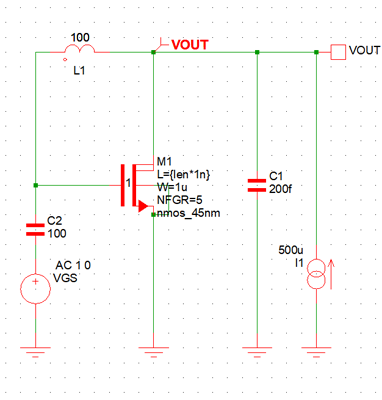The Choose Analysis configuration is shown below. The transistor length len is generated from in the Define List containing 45 and 90. These values are multiplied by 1n in the model definition for L={len*1n} (see M1 in the schematic).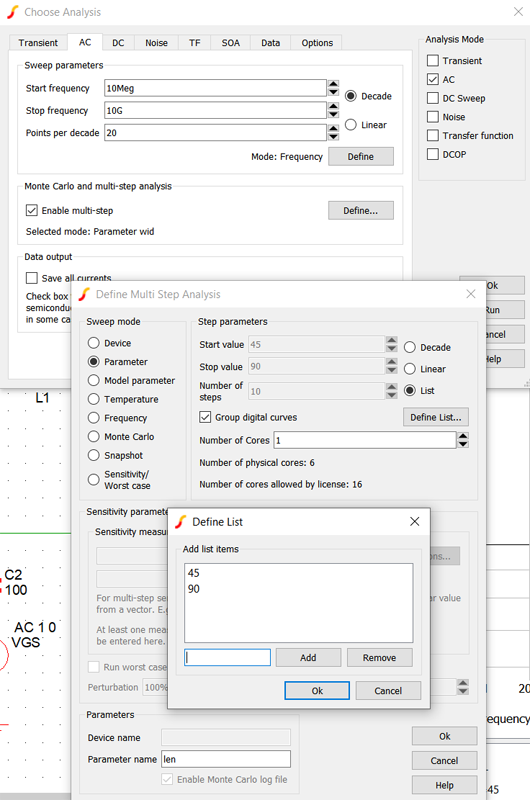The 3 dB bandwidth of the 90 nm NMOS transistor is 63.9 MHz and the 45 nm NMOS transistor is 453.3 MHz.The unity gain bandwidth of the 90 nm NMOS transistor is 941.7 MHz and the 45 nm NMOS transistor is 1307.8 MHz.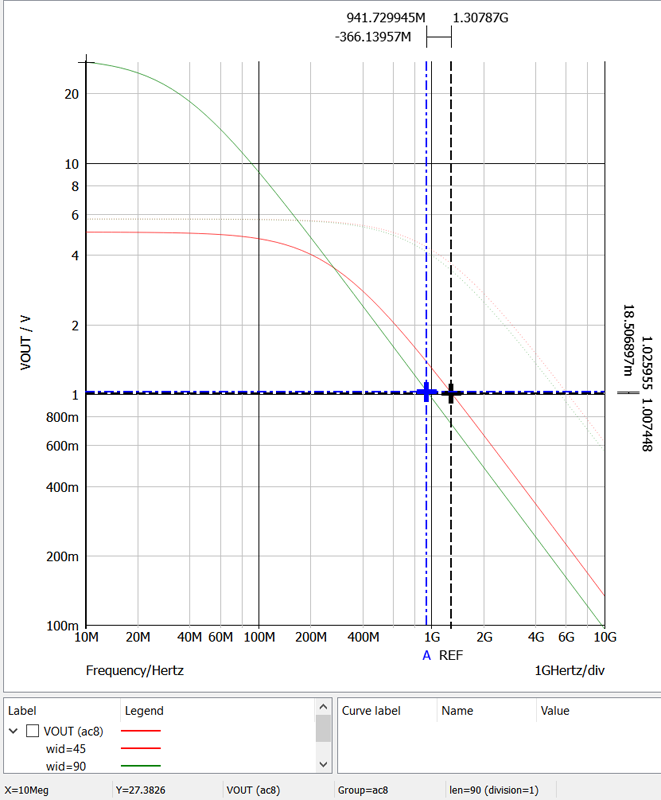### You may repeat these exercises for other model files available at: Model files

For example, the exercises can be repeated for SS/FF corners, PMOS devices, etc.

The exercise was recomputed for the pmos_45nm transistors since only the typical models for the 45 nm devices were available on the course website. The schematic and IV curve for the PMOS_45nm transistor is shown below. The parameters for the nmos-45nm transistor were used again for the pmos_45nm transistor (L=45n, W=1u, NFGR=5).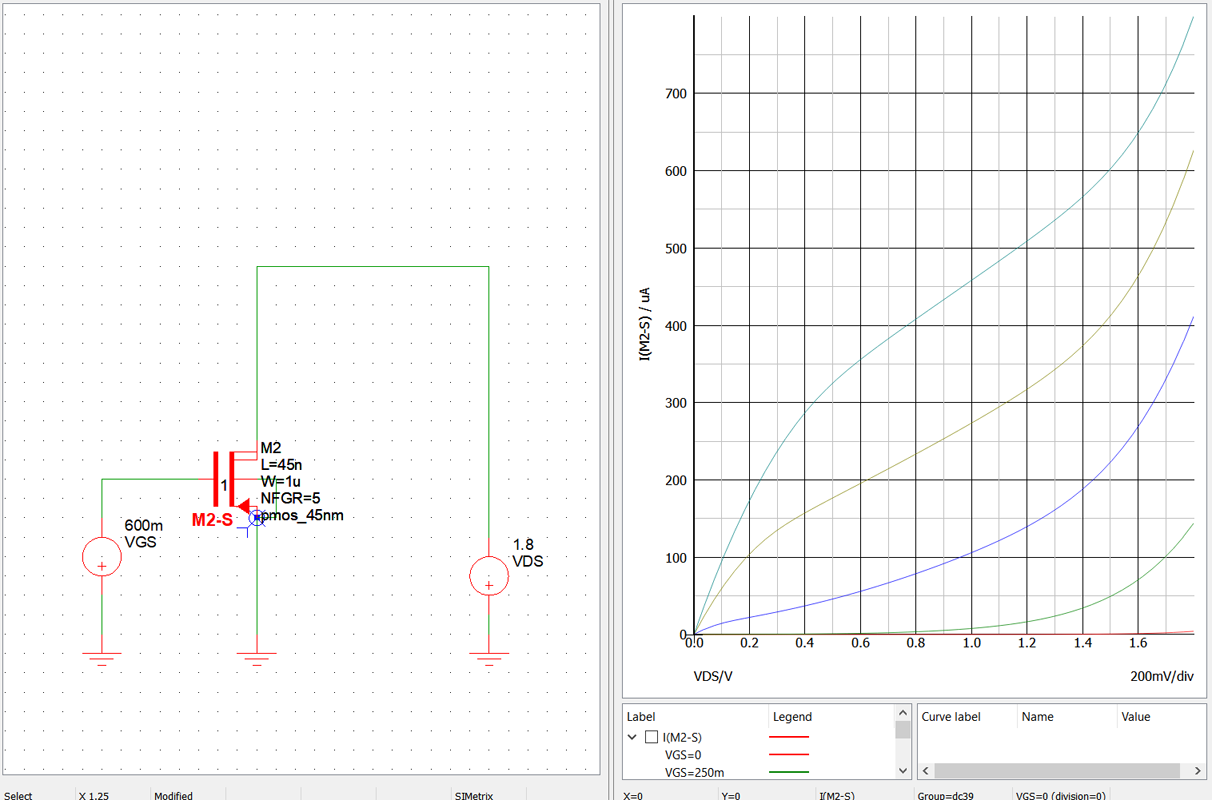The Choose Analysis DC VGS sweep of the PMOS_45nm transistor setup is shown in the figure below.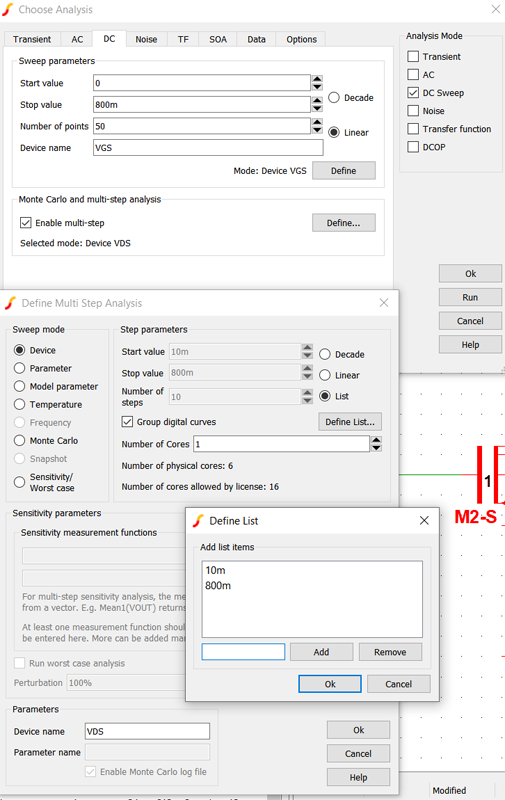Vt is estimated to be 400 mV at VDS=10mV using Id.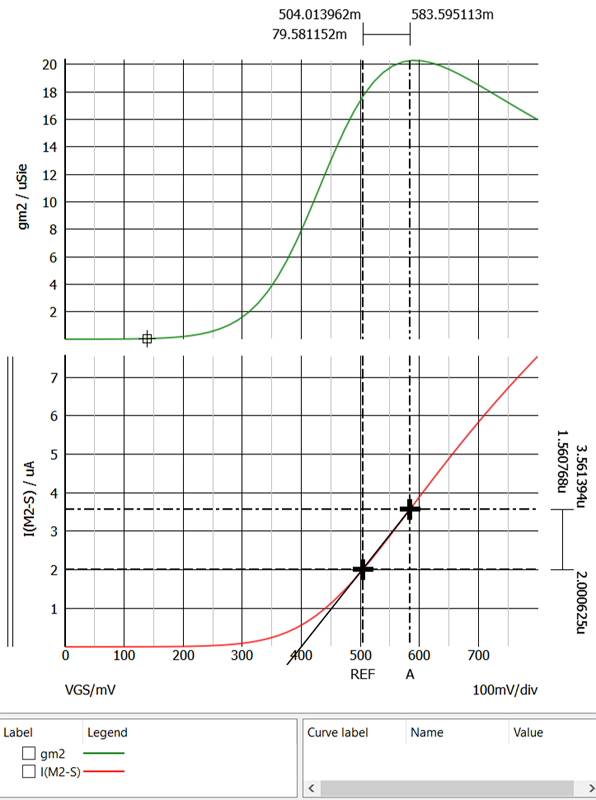Vt is estimated to be 215 mV at VDS=800 mV using gm. The lower Vt indicated DIBL is present in this device.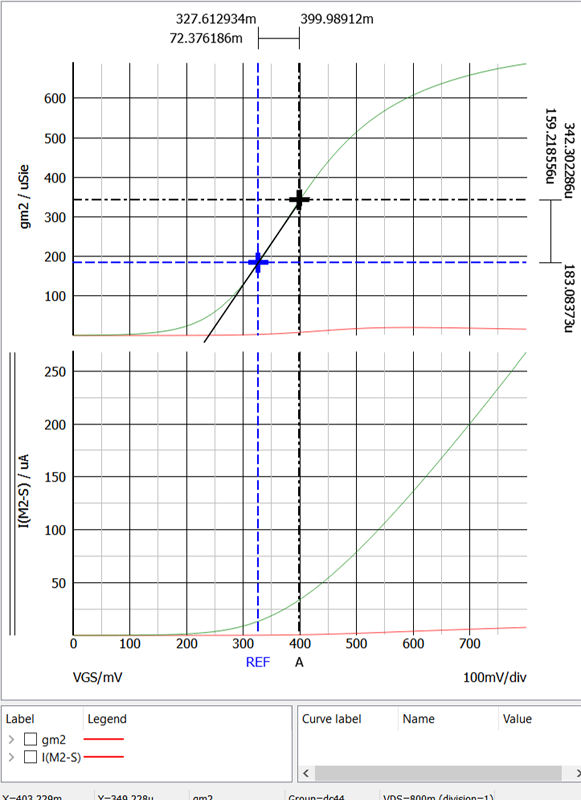The subthreshold slope is 105.7 mV/decade using Id at VDS=800 mV. n=1.78 was calculated using this subthreshold slope.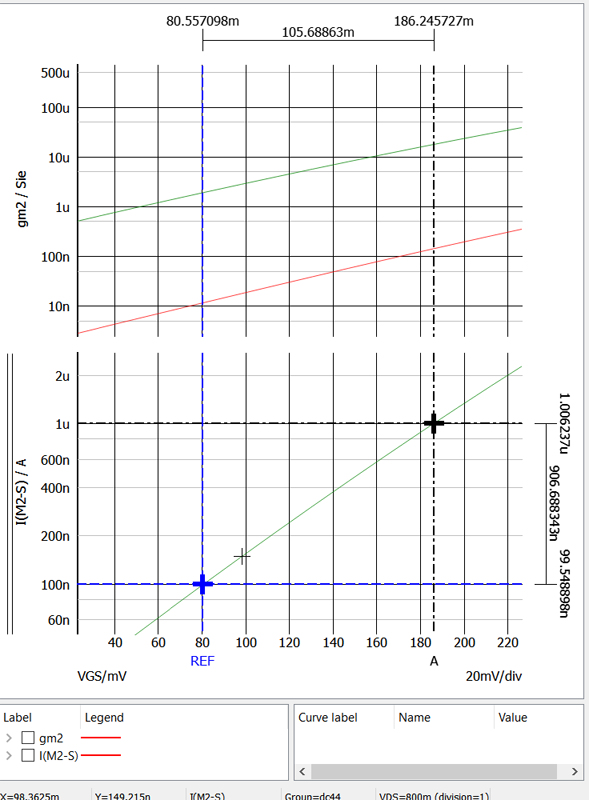Added the curve 1/diff(log10(m2#s)) to a new plot (top plot) to determine the subthreshold slope using
$$\frac{1}{\frac {\partial Log10(I_s)}{\partial V_{GS}}}$$
as shown in the Define Curve configuration shown below. In this PMOS transistor m2#d (Id) is negative so m2#s is the source current and is used to keep the plot value positive so that log scales can be used.Using the cursor on the green curve in the top plot, the subthreshold slope is 96.2752 mV/decade. This subthreshold slope yields n=1.62. Id does not increase at low VGS so GIBL isn't detected in this device.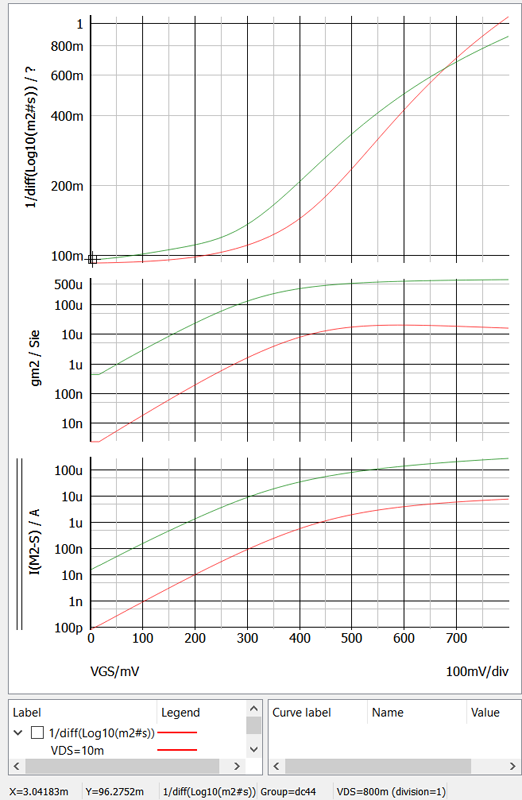From the AC plot below the intrinsic gain for the 45 nm PMOS transistors is 2.9 and the gain for the 90 nm NMOS transistor is 5.8 at 10 MHz. The unity gain bandwidth of the 90 nm PMOS transistor is 225.15 MHz and the 45 nm PMOS transistor is 563.69 MHz.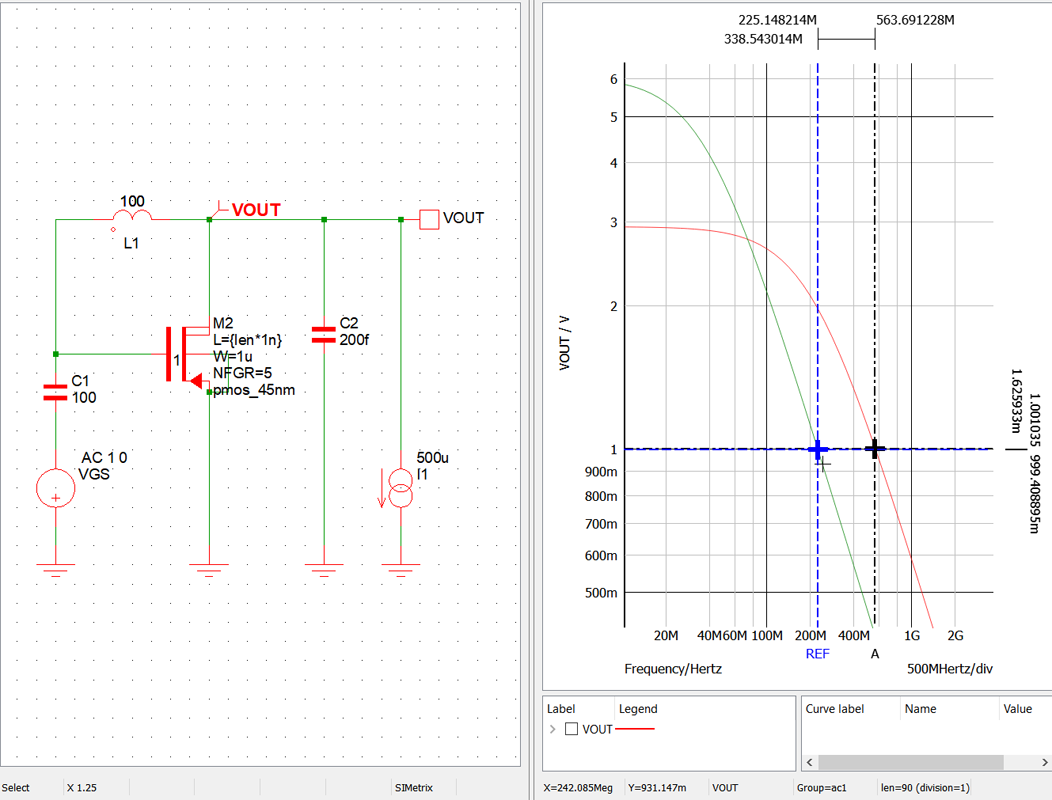### Summary

Summary of the transistor performance is shown in the table below.

 Parameter nmos_45nm L=90 nm nmos_45 L=45 nm pmos_45nm L=90 nm pmos_45nm L=45 nm Units Vt at VDS=10mv, Id ~hs~ 390 ~hs~ 400 mV Vt at VDS=800mv, gm ~hs~ 270 ~hs~ 215 mV Subthreshold at VDS=800mv, Id ~hs~ 100.5 ~hs~ 105.7 mV Subthreshold at VDS=800mv, gm ~hs~ 99.587 ~hs~ 96.3 mV n, at VDS=800mv, Id ~hs~ 1.69 ~hs~ 1.78 ~hs~ n at VDS=800mv, gm ~hs~ 1.67 ~hs~ 1.62 ~hs~ Gain at 10 MHz 27.4 5.0 5.8 2.9 ~hs~ Unity Gain Bandwidth 941.7 1307.8 225.2 563.7 MHz

## Acknowledgements

I really enjoyed this course. I appreciate Professor Carusone for teaching the course, Hooman Reyhani for his efforts organizing the course, and John Warner for providing the licenses for the SIMetrix Technologies simulator.

## Certificate